﻿ 中国碳捕获与封存技术的成本演化和技术扩散分析——-基于中国能源经济内生技术综合模型文章快速检索 高级检索

, 朱磊2, 范英2
1. 中国科学院大学 管理学院, 北京 100190;
2. 中国科学院 科技政策与管理科学研究所 能源与环境政策研究中心, 北京 100190

Analysis of cost and technological diffusion of carbon capture and storage for China—Based on Chinese energy economic endogenous technological model
DUAN Hong-bo1,2, ZHU Lei2, FAN Ying2
1. School of Management, University of Chinese Academy of Sciences, Beijing 100190, China;
2. Center for Energy and Environmental Policy, Institute of Policy and Management, Chinese Academy of Sciences, Beijing 100190, China
Abstract:In this paper, we explore the cost evolution and technological diffusion of CCS in the policy context by building an economy-environmental endogenous technological model. We find that CCS is difficult to get its long-term cost advantage, as compared to wind and nuclear, and the increasing of fuel cost should be responsible for this; Resource usage of CO2 could hedge against the use cost of CCS to some extent, while only the fuel cost is relatively stable, would this hedge effect be significant; The effect of CO2 resource usage on the penetration of CCS heavily depends on the CO2 amount used as resource, the introduction of subsidy policy will significantly improve this effect and promote CCS diffuse earlier.
Key words: endogenous technological model     carbon capture and storage     cost evolution     learning-by-doing     CO2 resource usage

0 引言

CCS技术对于中国具有特别的意义. 一方面,中国作为全球最大的新兴经济体,未来经济的大力发展还有赖于大量的能源投入. 同时,我们也是煤炭生产和消费大国,煤炭发电比率长期以来一直稳居70%以上,即使未来在提高能效和发展可再生能源方面做出了足够的努力,2030年时中国的煤炭消费占能源总消费的比重仍将超过50%. 因此,在相当长一段时期内,依靠高碳的煤炭来支撑中国经济发展的现状将难以改变; 另一方面,全球的气候变化形势正日益严峻,作为碳排放大国,未来中国势必将承担更多的自主减排任务,继而在减少碳排放和缓解气候变化方面作出应有的贡献. 由此可见,CCS技术将是能同时实现稳定经济发展和减少碳排放的重要技术选择.

CE3METL模型 (Chinese energy-economy-environmental model with endogenous technological change by employing logistic curves) 是中国单部门能源-经济-环境内生技术增长模型. CE3METL内生考虑基于经验学习的技术进步,并将能源技术划分碳基能源技术、低碳能源技术和无碳能源技术三类,其中碳基能源为煤炭、石油和天然气等化石能源; 低碳能源技术包括整体煤气化联合循环与碳捕获与封存技术组合技术(IGCC+CCS),传统粉煤发电技术与CCS组合技术 (PC+CCS); 而无碳能源技术主要包括水电、核电、生物质能和其它可再生能源技术. 模型结构见图 1.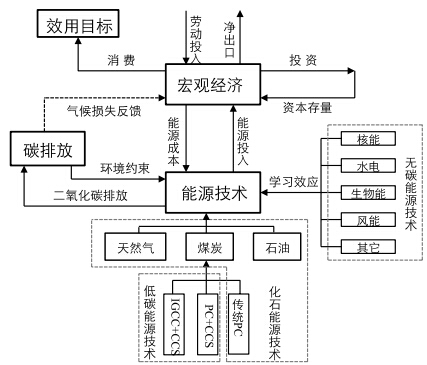图 1 CE3METL模型结构

CE3METL模型假设存在一个具有前瞻性的(for- ward-looking)中央计划人,它通过选择最优的投资、消费路径来最大化全社会的福利($Utility$). 此处,福利主要通过人均消费 ($c$) 来度量,因此,模型的目标函数可表示为:
 ${\rm Max}\sum_t\left(L(t)\cdot\log\left(\frac{c(t)}{L(t)}\right)\prod_{v=0}^{t}\left(1+\sigma(v)\right)^{-\Delta t}\right)$ (1)

 $\sigma(t)=\sigma_0\cdot{\rm e}^{-d_{\sigma}\cdot t}$ (2)
$d_{\sigma}$是时间偏好率的年递减因子. 1.1 经济模块

CE3METL生产过程主要通过科布-道格拉斯(Cobb-Douglas)型的常弹性替代生产函数来描述,投入因素包括资本($k_{t}$),劳动 ($L_{t}$) 以及能源 ($e_{t}$),即:
 $y(t)=(\alpha(t)\left(k(t)^{r} L(t)^{1-\gamma}\right)^{\rho}+\beta(t)e(t)^{\rho})^{\frac{1}{\rho}}$ (3)

 $k(t+1)=(1-\delta)k(t)+I(t+1)$ (4)

 $gdp(t)=y(t)-ec(t)$ (5)

 $ec(t)=e(t)pe(t)$ (6)

 $gdp(t)=I(t)+c(t)+x(t)-m(t)$ (1)

 $x(t)\ge\theta_{1} gdp(t)$ (8)
 $m(t)\le\theta_{2} gdp(t)$ (9)
1.2 能源技术模块

 $\frac{\mathrm{d}s_{i}(t)}{\mathrm{d}RP_{i}(t)}=a_{i}(t)s_{i}(t)\left(\hat{s}_{i}(t)\left(1+s_{i}(t)- \sum_{i} s_{i}(t)\right)-s_{i}(t)\right)$ (10)

 $R{P_i}(t) = \left\{\begin{array}{l} \dfrac{{{C_{\rm coal}}(t)\left( {1 + ta{x_{\rm coal}}(t)} \right)}}{{{C_i}(t)\left( {1 - su{b_i}(t)} \right)}}, {\rm{ }}\qquad\qquad i = {\rm{NUC,HYD,BIO,WIND,OTHER}} \\[2mm] \dfrac{{{C_{\rm coal}}(t)\left( {1 + ta{x_{\rm coal}}(t)} \right)}}{{{C_i}(t)\left( {1 + ta{x_i}(t)} \right)}}, {\rm{ }}\qquad\qquad i = {\rm{OIL,GAS}}\\[2mm] \dfrac{{{C_{\rm coal}}(t)\left( {1 + ta{x_{\rm coal}}(t)} \right)}}{{{C_i}(t)\left( {1 + ta{x_i}(t)} \right)\left( {1 - su{b_i}(t)} \right)}},{\rm{ }}\qquad i = {\rm{PC + CCS,IGCC + CCS}} \\ \end{array} \right.$ (11)

 $R_{i}^{\rm use}(t)=\lambda \frac{cu_{i}(t)C_{\rm oil}(t)}{cap_{i}(t)}\left(\frac{capr_{i}s_{i}(t)}{\sum\limits_{k} capr_{k}s_{k}(t)}\right), \quad\quad i=\mbox{PC+CCS,\ IGCC+CCS}$ (12)

 $cap_{i}(t)=capr_{i}s_{i}(t)e(t)$ (13)

CE3METL模型采用从价税率的形式来征收碳税,由于各种化石能源的碳排放是同质的,故而对单位CO$_{2}$所征收的税也应当是相等的. 据此,我们可根据煤炭的从价税率计算出石油、天然气以及两种低碳CCS技术的从价碳税税率,即:
 $tax_{i}(t)=\frac{tax_{\rm coal}emf_{i}C_{\rm coal}(t)}{emf_{\rm coal}C_{i}(t)},\qquad i=\mbox{OIL,\ GAS,\ PC+CCS,\ IGCC+CCS}$ (14)
$emf_{i}$表示技术$i$的排放因子. 于是,碳税$cart$ (每吨碳的价格)可以表示为:
 $cart(t)=\frac{tax_{\rm coal}(t)C_{\rm coal}(t)}{emf_{\rm coal}}=\frac{tax_{\rm oil}(t)C_{\rm oil}(t)}{emf_{\rm oil}}= \frac{tax_{\rm gas}(t)C_{\rm gas}(t)}{emf_{\rm gas}}$ (15)

 $C_{i}(t)=b_{i}KS_i(t)^{-lx_i}$ (16)

 $KS_{i}(t+1)=(1-\delta_{i})KS_{i}(t)+s_{i}(t+1)e(t+1)$ (17)

 $emis(t)=\left(\sum_{j} emf_{j}s_{j}(t)+\sum_{k} \left(1-capr_{k}\right)emf_{\rm coal}s_{k}(t)\right)e(t)$ (17)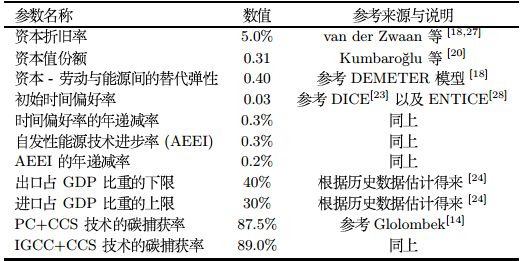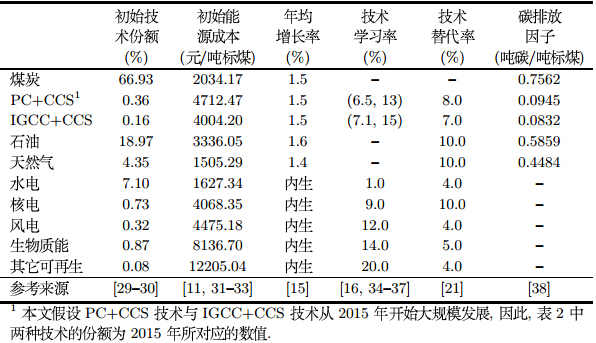McDonald等较早对各种新能源技术学习率进行了估计和对比,认为: 核能技术的学习率为5. 8%,风能和太阳能的学习率在不同国家、不同规模的风电厂有所不同,其中风能技术的学习率大体介于8%$\sim$18% 之间,而光伏太阳能技术则在20%左右. 此外,Mckinsey认为风能的技术学习率约为13% 左右,而太阳能则可达到23%. 据此,我们设定风能、核能、生物质能和其它可再生能源的学习率分别为12%、9%、14%和20%(表 2). 由于IGCC技术尚未大规模应用,因此暂时无法获得其相关技术数据,当前多用Flue-gas desulfurization (FGD)系统的运行数据来估计IGCC+CCS技术的替代学习率. Rubin等和Mckinsey分别估计IGCC+CCS技术的学习率为11% 和12%. Lohwasser等认为这一数值介于7. 1%$\sim$12. 2%之间,一般而言,仅考虑单因素学习效应时,学习率较低,而同时考虑基于研发和干中学"的双因素学习效应时,学习率普遍较高. 此外,Li等指出中国IGCC+CC电厂的投资成本学习率为9. 64%$\sim$20. 22%,发电成本学习率为7. 26%$\sim$14. 89%,而减排成本的学习率大致介于6. 36%$\sim$14. 62%之间. 综合考虑,CE3METL模型设定IGCC+CCS与PC+CCS技术的平均学习率分别为11. 1%和9. 8%(详见表 2). 3 结果与分析 3.1 情景设置

1. CO$_{2}$资源化利用在未来处置CO$_{2}$方面较有潜力,且能有效降低CCS技术的应用成本. 然而,中国目前还没有相关系统调查或估算以评估CO$_{2}$资源化利用的总体市场潜力.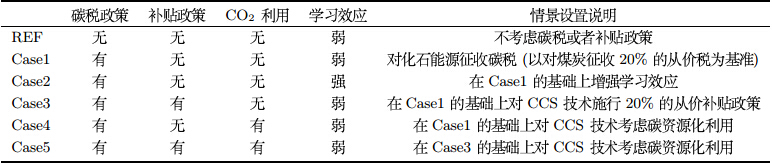3.2 政策背景下的CCS成本演化分析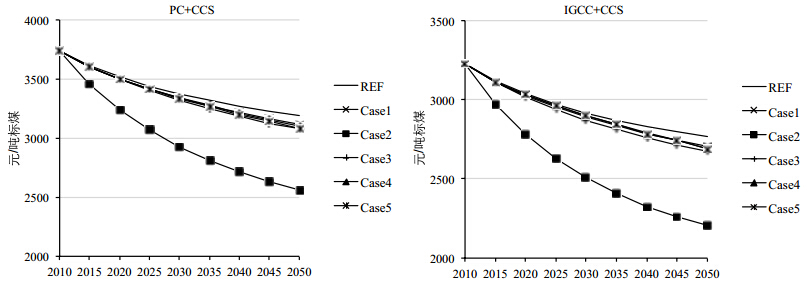图 2 不同情景下CCS技术成本的变化趋势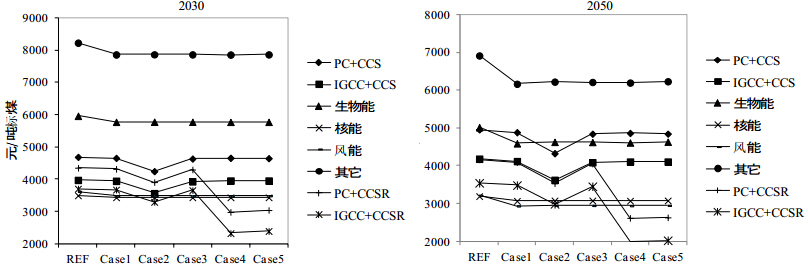图 3 2030年与2050年各种技术的使用成本对比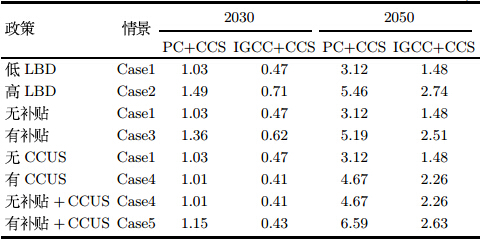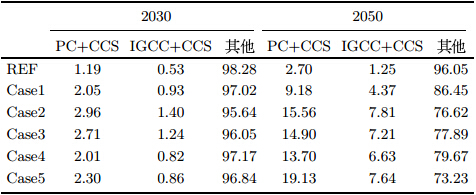4 结论与政策建议

  IPCC. Climate change 2007: Impacts, adaptation and vulnerability[R]. Cambridge: Cambridge University Press, 2007.  Willmott L R, Batchelder H R, Wenzell J L P, et al. Performance of a girbotol purification plant at Louisiana[R]. Department of the Interior, Bureau of Mines, 1956.  IEA/OECD. World energy outlook[R]. IEA/OECD, Paris, 2010.  IEA/OECD. Carbon capture and storage: Progress and next steps[R]. IEA/OECD, Paris, 2010.  IPCC. IPCC special report on renewable energy sources and climate change mitigation[R]. Cambridge: Cambridge University Press, 2010.  Liu H, Gallagher K. Driving carbon capture and storage forward in China[J]. Energy Procedia, 2009, 1(1): 3877-3884.  Edmonds J, Clarke J, Dooley J, et al. Stabilization of CO2 in a B2 world: Insights on the role of carbon capture and disposal, hydrogen, and transportation technologies[J]. Energy Economics, 2004, 26(4): 517-537.  Odenberger M, Johnsson F. The role of CCS in the European electricity supply system[J]. Energy Procedia, 2009, 1(1): 4273-428.  Schumacher K, Sands R. Greenhouse gas mitigation in a carbon constrained world-the role of CCS in Germany[J]. Energy Procedia, 2009, 1(1): 3755-3762.  Zhu L, Fan Y. A real options-based CCS investment evaluation model: Case study of China's power generation sector[J]. Applied Energy, 2011, 88(12): 4320-4333.  Cormos C C. Integrated assessment of IGCC power generation technology with carbon capture and storage (CCS)[J]. Energy, 2012, 42(1): 434-445.  Lilliestam J, Bielicki J M, Patt A G. Comparing carbon capture and storage (CCS) with concentrating solar power (CSP) Potential, costs, risks, and barriers[J]. Energy Policy, 2012, 47: 447-455.  Odenberger M, Johnsson F. Pathways for the European electricity supply system to 2050 —— The role of CCS to meet stringent CO2 reduction targets[J]. International Journal of Greenhouse Gas Control, 2010, 4: 327-340.  Golombek R, Greaker M, Kittelsen S A C, et al. Carbon capture and storage technologies in the European power market[J]. The Energy Journal, 2011, 32: 209-237.  Lohwasser R, Madlener R. Economics of CCS for coal plants: Impact of investment costs and efficiency on market diffusion in Europe[J]. Energy Economics, 2012, 34(3): 850-863.  van den Broek M, Hoefnagels R, Rubin E, et al. Effects of technological learning on future cost and performance of power plants with CO2 capture[J]. Progress in Energy and Combustion Science, 2009, 35(6): 457-480.  Riahi K, Rubin E S, Taylor M R, et al. Technological learning for carbon capture and sequestration technologies[J]. Energy Economics, 2009, 26: 539-564.  Gerlagh R, van der Zwaan B C C. Options and instruments for a deep cut in CO2 emissions: Carbon capture or renewable, taxes or subsidies?[J]. The Energy Journal, 2006, 27(3): 25-48.  气候组织. CCUS在中国: 18个热点问题[R]. 北京: 气候组织, 2011. The Climate Group. CCUS in China —— 18 hot issues[R]. Beijing: The Climate Group, 2011.  Kumbaroğlu G, Karali N, Arikan Y. CO2, GDP and RET: An aggregate economic equilibrium analysis for Turkey[J]. Energy Policy, 2008, 36(7): 2694-2708.  Anderson D, Winne S. Innovation and threshold effects in technology responses to climate change[R]. Working Paper 43, Tyndall Centre for Climate Change Research, 2003.  Barreto L, Kypreos S. Endogenizing R&D and market experience in the "bottom-up" energy-systems ERIS model[J]. Technovation, 2004, 24(8): 615-629.  Nordhaus W D, Boyer J. Warming the world: Economic models of global warming[M]. Cambridge: MIT Press, 2000.  国家统计局. 中国统计年鉴2011[M]. 北京: 中国统计出版社, 2012. State Statistics Bureau. Chinese statistical yearbook 2011[M]. Beijing: China Statistical Publishing House, 2012.  门可佩, 官琳琳, 尹逊震. 基于两种新型灰色模型的中国人口预测[J]. 经济地理, 2007, 27(6): 942-945. Men Kepei, Guan Linlin, Yin Xunzhen. Prediction of China's population based on two new grey models[J]. Economic Geography, 2007, 27(6): 942-945.  World Bank. International trade and climate change[R]. Washington DC: World Bank, 2007.  van der Zwaan B C C, Gerlagh R, Klaassen G, et al. Endogenous technological change in climate change modeling[J]. Energy Economics, 2002, 24(1): 1-19.  Popp D. ENTICE: Endogenous technological change in the DICE model of global warming[J]. Journal of Environmental Economics and Management, 2004, 48(1): 742-768.  国家统计局. 中国能源统计年鉴2011[M]. 北京: 中国统计出版社, 2012. State Statistics Bureau. Chinese energy statistical yearbook 2011[M]. Beijing: China Statistical Publishing House, 2012.  国家电力监管委员会.中国电力监管年度报告 2011[R/OL]. http://www.serc.gov.cn, 2012. State Electricity Regulatory Commission. Annual report of China power regulation 2011[R/OL]. http://www.serc. gov.cn, 2012.  张建府. 碳捕集与封存技术 (CCS) 成本及政策分析[J]. 中外能源, 2010, 3: 21-25. Zhang Jianfu. Analysis of cost and policies for CCS[J]. Sino-Global Energy, 2010, 3: 21-25.  张斌, 倪维斗, 李政. 火电厂和IGCC及煤气化SOFC混合循环减排CO2的分析[J]. 煤炭转化, 2005, 28(11): 1-7. Zhang Bin, Ni Weidou, Li Zheng. Analysis of conventional power plant, IGCC and coal gasification SOFC hybrid with CO2 mitigation[J]. Coal Conversion, 2005, 28(11): 1-7.  2050中国能源和碳排放研究课题组(2050能源报告). 2050中国能源和碳排放报告[R]. 北京: 科学出版社, 2009. 2050 China Energy and CO2 Emissions Research Group (2050 Energy Group). 2050 China energy and CO2 emissions report[R]. Beijing: Science Press, 2009.  McDonald A, Schrattenholzer L. Learning rates for energy technologies[J]. Energy Policy, 2001, 29(4): 255-261.  McKinsey. Carbon capture and storage: Assessing the economics[R]. New York: McKinsey & Company, Inc, 2008.  Rubin E S, Taylor M R, Yeh S, et al. Learning curves for environmental technologies and their importance for climate policy analysis[J]. Energy, 2004, 29(9-10): 1551-1559.  Li S, Zhang X S, Gao L, et al. Learning rates and future cost curves for fossil fuel energy systems with CO2 capture: Methodology and case studies[J]. Applied Energy, 2012(93): 348-356.  IPCC. Guidelines for national greenhouse gas inventories[R]. Japan: Institute for Global Environmental Strategies, 2006.
0

#### 文章信息

DUAN Hong-bo, ZHU Lei, FAN Ying

Analysis of cost and technological diffusion of carbon capture and storage for China—Based on Chinese energy economic endogenous technological model

Systems Engineering - Theory & practice, 2015, 35(2): 333-341.